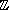# OEF modular arithmetic --- Introduction ---

This module actually contains 23 exercises on computations in the finite ring/n.

Consider a map , which sends to . Fill the following table for by dragging the numbers given below.

### Cubic fill

Consider a map , which sends to . Fill the following table for by dragging the numbers given below.

### Division fill

Consider a map , which sends to . Fill the following table for by dragging the numbers given below.

### Division I

Compute / in/. The result must be represented by a number between 0 and .

### Division II

Compute / in/. The result must be represented by a number between 0 and .

### Division III

Compute / in/. The result must be represented by a number between 0 and .

### Zero divisors

Is a zero divisor in/?

### Zero divisor II

Find the set of zero divisors in/. (In this exercise we don't consider 0 as a zero divisor.)

Write each element by a number between 1 and , and separate the elements by commas.

### Zero divisors III

We have =2, where is a prime. How many zero divisors there are in/?

In this exercise we don't consider 0 as a zero divisor.

### Inverse I

Find the inverse of in/. The result must be represented by a number between 0 and .

### Inverse II

Find the inverse of in/. The result must be represented by a number between 1 and .

### Inverse III

Find the inverse of in/. The result must be represented by a number between 0 and .

### Invertible power

is a prime. Consider the function f:/->/defined by f(x)=x .

Is f bijective?

### Multiplication fill

Consider a map , which sends to . Fill the following table for by dragging the numbers given below.

### Polynomial fill

Consider a map , which sends to . Fill the following table for by dragging the numbers given below.

### Powers

Compute the element in/. The result must be represented by a number between 0 and .

### Powers II

is a prime number. Compute the element in/. The result must be represented by a number between 0 and .

### Power fill

Consider a map , which sends to . Fill the following table for by dragging the numbers given below.

### Roots

is a prime number. There is an element a in/, such that a is congruent to modulo . Find a.

The result must be represented by a number between 0 and .

### Simple computations modulo n

Compute in/. The result must be represented by a number between 0 and .

### Squares

Find the set of squares in/. (A square in/is an element which is the square of another one.)

Write each element by a number between 0 and , and separate the elements by commas.

### Sum and product

Find two integers , such that

0, 0,

+(mod ) , ×(mod ) .

You may enter the two numbers in any order.

### Trinomial fill

Consider a map , which sends to . Fill the following table for by dragging the numbers given below.

Other exercises on: modular arithmetics

In order to access WIMS services, you need a browser supporting forms. In order to test the browser you are using, please type the word wims here: and press Enter''.